# College Chemistry : Molecules and Compounds

## Example Questions

### Example Question #1 : Orbital Hybridization

What is the orbital hybridization for nitrogen in the molecule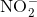?

sp

sp3

sp2

sp3d

sp2

Explanation:

Start by drawing the Lewis structure ofhasvalence electrons.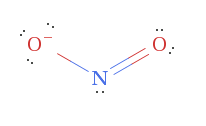Since the nitrogen hasoxygen atoms bonded to it and a lone pair, the steric number must be. From that, we know that it must have sp2 hybridization.

### Example Question #2 : Orbital Hybridization

Which of the following types of hybridization is found in the molecule shown below?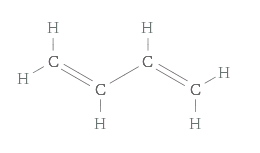sp3

sp2

sp3d

sp

sp2

Explanation:Look at each of the carbon atoms in the chain. Each carbon atom hasother atoms bonded to it. This means that the steric number of each carbon is. Thus, the only type of hybridization found in this molecule is sp2.

### Example Question #3 : Orbital Hybridization

What is the hybridization for the atom circled in red?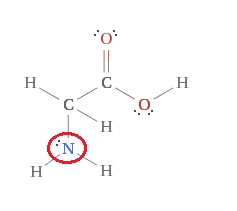sp2

sp3d

spsp3sp3

Explanation:Start by counting the number of atoms the nitrogen is bonded to. It hasbonded atoms. The nitrogen also has a lone pair. This means the steric number of the nitrogen is. Thus, it must have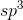hybridization.

### Example Question #4 : Orbital Hybridization

Dimethylsulfoxide is can be drawn with the following Lewis structure: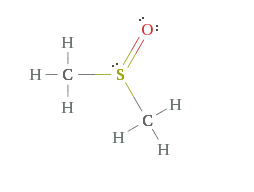What is the hybridization about the sulfur atom?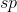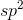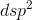Explanation:Notice that there aregroups bonded to the sulfur. The groups are the double bond to the oxygen, the lone pair, and the bonds to the two separate carbons.

Since there aregroups on the sulfur, it must havehybridization.

### Example Question #5 : Orbital Hybridization

What is the hybridization of selenium in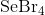?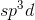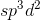Explanation:

Start by drawing the Lewis structure.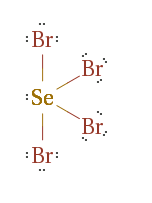Notice that selenium hasgroups around it: 4 bromines and 1 lone pair.

Molecules withelectron groups will exhibithybridization.

### Example Question #6 : Orbital Hybridization

What kind of hybridization would be expected of the carbon atom involved in a ketone group?No hybridization would be expectedExplanation:

For this question, we're asked to identify which kind of hydridization, if any, a carbonyl carbon of a ketone group will have.

First, let's recall that a ketone group is a functional group in which a carbon atom contains a double bond to an oxygen atom. In addition, the carbon also has a single bond to each of two other atoms, neither of which are hydrogen.

Let's also recall that a double bond consists of one sigma bond and one pi bond. Each of the two single bonds that carbon has to the other atoms will also be sigma bonds, because these are both single bonds. Thus, in total, this carbon atom will have three sigma bonds and one pi bond.

In order to have a pi bond, there must be p orbital overlap. Thus, one out of the three p orbitals from the carbon will be used towards contributing to the pi bond with the oxygen. Consequently, we have one s orbital and two p orbitals left over. These three orbitals can hybridize to become threeorbitals. Each of theseorbitals will take place in the three sigma bonds that the carbon atom has.

### Example Question #7 : Orbital Hybridization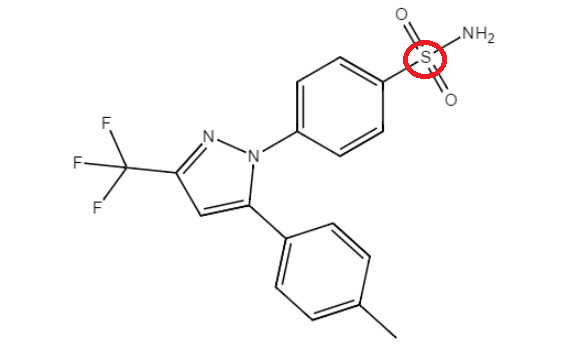What is the hybridization of the circled sulfur atom in the given molecule?Explanation:Count the number of groups that the sulfur has attached to it. It is attached to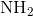, two oxygen atoms, an done carbon. Since there are four groups attached to the sulfur, it must havehybridization.

### Example Question #8 : Orbital Hybridization

What is the hybridization found around the nitrogen atom in nitrous acid?

The hybridization of the nitrogen atom cannot be determined.Explanation:

Start by recalling that nitrous acid is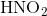.

Next, draw its Lewis Structure: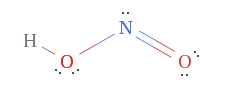Notice that the nitrogen is bonded to two oxygen atoms. The nitrogen atom also has a lone pair. Since there aregroups attached to the nitrogen, it must havehybridization.

### Example Question #9 : Orbital Hybridization

Ethylene is a hydrocarbon with the molecular formula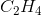. What is the orbital hybridization and orbital orientation of the Carbon atoms in ethylene?; trigonal planar; tetrahedral; tetrahedral; trigonal planar; trigonal planar

Explanation:

In ethylene, the two central Carbon atoms are bonded together with a double covalent bond. In order to form a double bond, Carbon always takes onhybridization. The double bond consists of a sigma bond caused by the overlapping of a pair ofhybridized orbitals and a pi bond caused by the overlapping of neighboring unhybridizedorbitals. Further,hybridization always results in hybrid orbitals that are oriented in a trigonal planar manner, with a separation angle of 120° for maximum electron separation between the three sigma-bondingorbitals. Thus, all other answers are incorrect.

### Example Question #10 : Orbital Hybridization

In the molecule hydrogen cyanide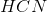, what is the orbital hybridization of the carbon atom?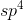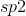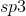In HCN, Carbon is bonded to Nitrogen with a triple covalent bond consisting of one sigma bond and two pi bonds. The sigma bond is formed by overlappinghybridized orbitals, with the two remaining unhybridizedorbitals overlapping to form the two pi bonds.